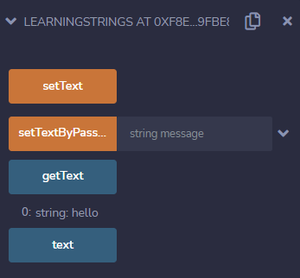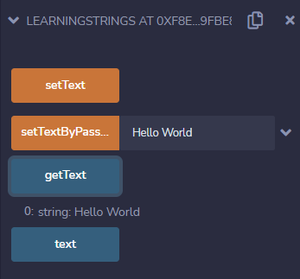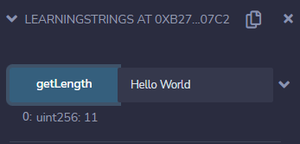Open in App
Not now

# Solidity – Strings

• Difficulty Level : Basic
• Last Updated : 04 Jul, 2022

Solidity is syntactically similar to JavaScript, C++, and Python. So it uses similar language structures to those languages. Strings in Solidity is a data type used to represent/store a set of characters.

Examples:

“Hii” // Valid string

“Hello World” // Valid string

“2022” // Valid string

In Solidity data types are classified into two categories: Value type and Reference type

• Strings in Solidity is a reference type of data type which stores the location of the data instead of directly storing the data into the variable.
• They are dynamic arrays that store a set of characters that can consist of numbers, special characters, spaces, and alphabets.
• Strings in solidity store data in UTF-8 encoding.
• Like JavaScript, both Double quote(” “) and Single quote(‘ ‘) can be used to represent strings in solidity.

“Hello World”  // Valid string

‘Hello World’  // Valid string

‘Hello World”  // Invalid string

Example 1: Below is the solidity program to assign value to string variable using a function:

## Solidity

 `// Solidity program to assign value  ` `// to string variable using function ` `pragma solidity ^0.5.0; ` ` `  `contract` `LearningStrings  ` `{ ` `   ``string text; ` `   `  `   ``function setText () ``public` `returns (string memory)  ` `   ``{ ` `     ``text = ``"Hello World"``; ` `     ``return` `text; ` `   ``} ` `}`

Explanation:

In the above code, the setText function is used to assign “Hello World” to string text.

Example 2: The strings can be initialized with a value in solidity by passing strings to function or by assigning the strings with a text directly. Below is the solidity program to assign value to a string variable:

## Solidity

 `// Solidity program to set string value  ` `// using function with parameters ` `pragma solidity ^0.5.0; ` ` `  `contract` `LearningStrings ` `{ ` `    ``string ``public` `text; ` `  `  `    ``// Assigning the text directly ` `    ``function setText() ``public`  `    ``{ ` `        ``text = ``'hello'``; ` `    ``} ` ` `  `    ``// Assigning the text by passing the value in the function ` `    ``function setTextByPassing(string memory message) ``public`  `    ``{ ` `        ``text = message; ` `    ``} ` ` `  `    ``// Function to get the text ` `    ``function getText() view ``public` `returns (string memory)  ` `    ``{ ` `        ``return` `text; ` `    ``} ` `}`

Output:setText()setTextByPassing()

### Escape Characters

Additionally, string literals also support the following escape characters:

### Length Of String

Strings in Solidity do not have a length method to find the character count in strings, but we can convert the string to bytes and get its length. In solidity, we can convert Strings to bytes and vice versa.

Examples:

To convert string to bytes:

string text = “Hii”;
bytes b = bytes(text);

To convert bytes to string:

bytes memory b = new bytes(5);
string text = string(b);

Below is the solidity program to find the length of the string:

## Solidity

 `// Solidity program to get  ` `// length of a string ` `pragma solidity ^0.5.0; ` ` `  `contract` `LearningStrings  ` `{ ` `   ``function getLength(string memory s) ``public` `pure returns (uint256)  ` `   ``{ ` `        ``bytes memory b = bytes(s); ` `        ``return` `b.length; ` `    ``} ` `}`

Output:My Personal Notes arrow_drop_up
Related Articles